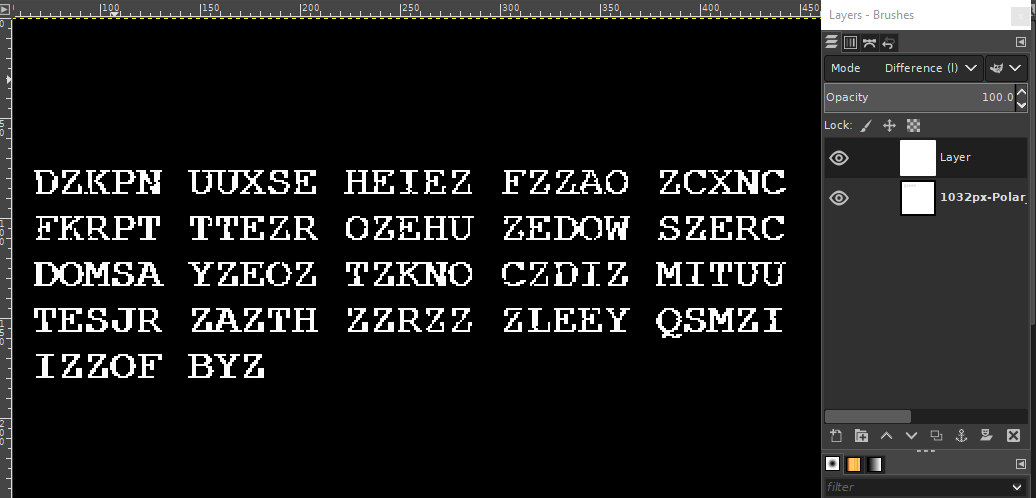# Mark Clowes

## Index - 2013 Xmas Cipher Challenge Part 3 Solution

2018-05-24This was a tricky one, and I still don't know if I solved it in the expected way. I will only link the file as it is quite big:

[challenge3.png]

So, that is all we have to work with at this point. A picture of a polar bear. "The location of the message has been forgotten." I recall checking for PNG comments and so on and didn't find anything. The one thing that stands out is the use of PNG.

PNG files are lossless. This is great for certain web graphics, not so great for photos as compression can be poor. But PNG would be required if there were information hidden in a photograph that would be destroyed if lossily compressed with for example JPEG.

So I fed the picture of a polar bear into a reverse image search engine and found the original:

Eye-balling it they do look indistinguishable, but let's load it up in GIMP.

If there has been any subtle edits we should be able to pick them out by loading up both images as layers with the top layer in "Difference" mode. Then some fuzzy 0-threshold selection and inversion... Success! We've got some cipher text:``````DZKPN UUXSE HEIEZ FZZAO ZCXNC
FKRPT TTEZR OZEHU ZEDOW SZERC
DOMSA YZEOZ TZKNO CZDIZ MITUU
TESJR ZAZTH ZZRZZ ZLEEY QSMZI
IZZOF BYZ``````

Okay, we're half way there. Let's do a frequency analysis:

``````Z   25
E   11
O   7
T   7
I   5
R   5
S   5
U   5
C   4
D   4
A   3
F   3
H   3
K   3
M   3
N   3
Y   3
P   2
X   2
B   1
J   1
L   1
Q   1
W   1
G   0
V   0``````

In English, E is the most commonly used letter. If a substitution cipher is in use this can be a good starting point; the most common letter in your cipher text is probably E substituted.

However Z is by far the most common in this cipher text. There is one character that is more common than E of course: space. So this shows that there is probably no substitution, and spaces are represented with Z. This is a good candidate for a column cipher, which did indeed turn out to be correct.

A column cipher is an ancient method that was quite literally about wrapping your cipher text on paper around a correctly sized column, and then reading along it. The key is the size of the column.

So for our cipher text that means rearranging the cipher text vertically, and then starting a new column. It should be clear from looking at the answer how it was achieved. This was found by trying various column sizes:

``````D   E   A   R   Z   S   A   N   T   A   Z   I
Z   H   O   P   E   Z   Y   O   U   Z   L   I
K   E   Z   T   H   E   Z   C   U   T   E   Z
P   I   C   T   U   R   E   Z   T   H   E   Z
N   E   X   T   Z   C   O   D   E   Z   Y   O
U   Z   N   E   E   D   Z   I   S   Z   Q   F
U   F   C   Z   D   O   T   Z   J   R   S   B
X   Z   F   R   O   M   Z   M   R   Z   M   Y
S   Z   K   O   W   S   K   I   Z   Z   Z   Z``````

And with the Z's removed:

``````D   E   A   R       S   A   N   T   A       I
H   O   P   E       Y   O   U       L   I
K   E       T   H   E       C   U   T   E
P   I   C   T   U   R   E       T   H   E
N   E   X   T       C   O   D   E       Y   O
U       N   E   E   D       I   S       Q   F
U   F   C       D   O   T       J   R   S   B
X       F   R   O   M       M   R       M   Y
S   Z   K   O   W   S   K   I                ``````

PrevNext